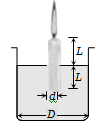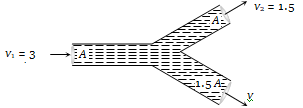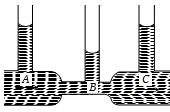A boat carrying steel balls is floating on the surface of water in a tank. If the balls are thrown into the tank one by one, how will it affect the level of water ?

(a) It will remain unchanged

(b) It will rise

(c) It will fall

(d) First it will first rise and then fall

Concept Questions :-

Equation of continuity

Difficulty Level:

Two pieces of metal when immersed in a liquid have equal upthrust on them; then

(a) Both pieces must have equal weights

(b) Both pieces must have equal densities

(c) Both pieces must have equal volumes

(d) Both are floating to the same depth

Concept Questions :-

Archimedes principle
High Yielding Test Series + Question Bank - NEET 2020

Difficulty Level:

A candle of diameter d is floating on a liquid in a cylindrical container of diameter D (D>>d) as shown in figure. If it is burning at the rate of 2cm/hour then the top of the candle will(a) Remain at the same height

(b) Fall at the rate of 1 cm/hour

(c) Fall at the rate of  2 cm/hour

(d) Go up the rate of 1cm/hour

Concept Questions :-

Archimedes principle
High Yielding Test Series + Question Bank - NEET 2020

Difficulty Level:

Construction of submarines is based on
(a) Archimedes’ principle                (b) Bernoulli’s theorem
(c) Pascal’s law                              (d) Newton’s laws

Concept Questions :-

Archimedes principle

Difficulty Level:

In which one of the following cases will the liquid flow in a pipe be most streamlined  ?

(a) Liquid of high viscosity and high density flowing through a pipe of small radius

(b) Liquid of high viscosity and low density flowing through a pipe of small radius

(c) Liquid of low viscosity and low density flowing through a pipe of large radius

(d) Liquid of low viscosity and high density flowing through a pipe of large radius

Concept Questions :-

Types of flow
High Yielding Test Series + Question Bank - NEET 2020

Difficulty Level:

Two water pipes of diameters 2 cm and 4 cm are connected with the main supply line. The velocity of flow of water in the pipe of 2 cm diameter is

(a) 4 times that in the other pipe

(b) times that in the other pipe

(c) 2 times that in the other pipe

(d) times that in the other pipe

Concept Questions :-

Equation of continuity
High Yielding Test Series + Question Bank - NEET 2020

Difficulty Level:

An incompressible liquid flows through a horizontal tube as shown in the following fig. Then the velocity v of the fluid is(a)    3.0 m/s                           (b) 1.5 m/s

(c) 1.0 m/s                             (d) 2.25 m/s

Concept Questions :-

Equation of continuity
High Yielding Test Series + Question Bank - NEET 2020

Difficulty Level:

The velocity of kerosene oil in a horizontal pipe is 5 m/s. If $\mathrm{g}=10\mathrm{m}/{\mathrm{s}}^{2}$ then the velocity head of oil will be
(a) 1.25 m                                (b) 12.5 m
(c) 0.125 m                              (d) 125 m

Concept Questions :-

Equation of continuity
High Yielding Test Series + Question Bank - NEET 2020

Difficulty Level:

In the following fig. is shown the flow of liquid through a horizontal pipe. Three tubes A, B and C are connected to the pipe. The radii of the tubes A, B and C at the junction are respectively 2 cm, 1 cm and 2 cm. It can be said that the(a) Height of the liquid in the tube A is maximum

(b) Height of the liquid in the tubes A and B is the same

(c) Height of the liquid in all the three tubes is the same

(d) Height of the liquid in the tubes A and C is the same

Concept Questions :-

Bernoulli theorem
High Yielding Test Series + Question Bank - NEET 2020

Difficulty Level:

A manometer connected to a closed tap reads . When the valve is opened, the reading of manometer falls to  , then velocity of flow of water is
(a) 100 m/s                                       (b) 10 m/s
(c) 1 m/s                                           (d) $10\sqrt{10}$ m/s

Concept Questions :-

Bernoulli theorem